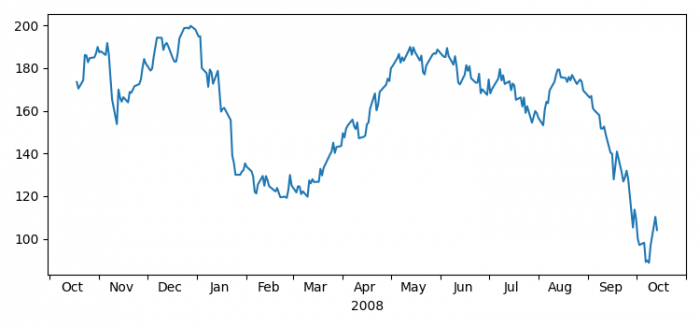# Centering x-tick labels between tick marks in Matplotlib

MatplotlibPythonData Visualization

To place labels between two ticks, we can take the following steps−

• Load some sample data, r.
• Create a copy of the array, cast to a specified type.
• Create a figure and a set of subplots using subplots() method.
• Plot date and r sample data.
• Set the locator of the major/minor ticker using set_major_locator() and set_minor_locator() methods.
• Set the locator of the major/minor formatter using set_major_locator() and set_minor_formatter() methods.
• Now, place the ticklabel at the center.
• To display the figure, use show() method.

## Example

import numpy as np
import matplotlib.cbook as cbook
import matplotlib.dates as dates
import matplotlib.ticker as ticker
import matplotlib.pyplot as plt
plt.rcParams["figure.figsize"] = [7.00, 3.50]
plt.rcParams["figure.autolayout"] = True
with cbook.get_sample_data('aapl.npz') as fh:
r = r[-250:]
date = r.date.astype('O')
fig, ax = plt.subplots()
ax.xaxis.set_major_locator(dates.MonthLocator())
ax.xaxis.set_minor_locator(dates.MonthLocator(bymonthday=15))
ax.xaxis.set_major_formatter(ticker.NullFormatter())
ax.xaxis.set_minor_formatter(dates.DateFormatter('%b'))
for tick in ax.xaxis.get_minor_ticks():
tick.tick1line.set_markersize(0)
tick.tick2line.set_markersize(0)
tick.label1.set_horizontalalignment('center')
imid = len(r) // 2
ax.set_xlabel(str(date[imid].year))
plt.show()

## Output